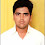## Saturday, August 30, 2014

### Send expression to function using Expression class

This trick is very useful when we want to execute different expressions within one single function where the function argument will remain unchanged.

The best example of the situation is Repository design pattern.  In Repository pattern, we can create single generic function, which will take expression as argument and execute on some context class. As per expression the query, will get generate and execute.

The Advantage of the method is that single generic function can able to server many expression. We will implement it shortly.

Let’s understand the class at first. The prototype of the class is

public sealed class Expression<TDelegate> : LambdaExpression

Expression class has two version one concrete class “Expression” and another is “Expression<TDelegate>”  . In this example we will use the generic version of the class. Here we are seeing that Expression is taking TDelegate.

So, let’s implement example. Here is the simple model class which we will use in this class.

public class Person
{
public Int32 Id { get; set; }
public string name {get;set;}
public string surname{get;set;}
}

Here is the context class of the application. We have inherit it from DbContext class.

public class DataContext :DbContext
{
public DataContext() : base("ApplicationConn") { }

public DbSet<Person> PersonEntity { get; set; }
}

Let us generate database and migrate. Once we seed database it will create below table.

Now, let’s create one class and function which will take Expression as argument. Here is sample code.

public class myClass<T> where T : Person
{
public IDbSet<T> dbSet = new DataContext().Set<T>();

public IEnumerable<Person> Fun(Expression<Func<T, bool>> where)
{
var person = dbSet.Where(where).ToList();
return person;
}
}

We are seeing that Fun function is taking Expression as argument. The argument can be any expression, for example, LINQ query. We are using The Context class to fetch data from Database. Now, we will call the function and send argument. Have a look on below code.

class Program
{
static void Main(string[] args)
{

var myClass = new myClass<Person>();

var person = myClass.Fun(f => f.Id == 1).ToList();
Console.WriteLine("Person with Id =1 \n");
Console.WriteLine(person.name + " " + person.surname + "\n\n");

var persons = myClass.Fun(f => f.Id == 1 || f.surname == "Kumar").ToList();
Console.WriteLine("Person whose Id = 1 and surname =Kumar");

Console.WriteLine(persons.name + " " + persons.surname);
Console.WriteLine(persons.name + " " + persons.surname);

}
}

In first call we are sending one expression which will fetch person based on Id.  We are passing Id=1 and it will fetch person whose Id is 1.
In second class, we are fetching all person whose Id is 1 or surname is “Kumar” and obviously it will return two persons.
Here is sample output.

#### 1 comment:

1.Can we create dynamic queries like Name starts with,date in between,...on fly..
I mean not like ID and Surname we have two implementations for these two ...